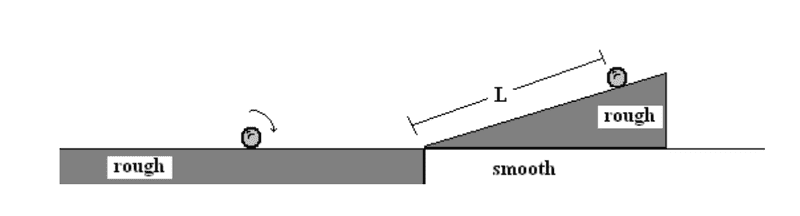# Rotational Inertia Physics C1. Homework Statement

A solid sphere (mass of m, radius of r and I=2/5 mr2) is rolling without slipping on
a rough surface with a speed of v. A ramp (mass of 2m and angle of θ) rests on a
smooth surface and is free to slide on the surface. As the ball rolls up the ramp, the
ramp begins to move. Provide all answers in terms of the given variables and any
fundamental constants.

A. What will be the speed of the ball/ramp when the ball reaches its highest point on
the ramp?

B. What distance L will the ball roll up the incline?

C. What will be the speeds of the ball and the ramp after the ball rolls back down off
of the ramp?

## Homework Equations

Conservation of Momentum, Conservation of Energy

## The Attempt at a Solution

A. mv=(m+2m)v
mv=3mv
vf=1/3v

B.
we now have vf which is v/3 we can solve

ΣEi=1/2 Iω2+1/2mv2=

1/2(2/5mr2)(v/r)2+1/2mv2=

1/5mr2+1/2mv2=7/10mv2

ΣEf=mgh+1/2*3mvf2=

mgh+1/2*3m(v/3)2=

mgh+1/6mv2=7/10 mv2

h=8v2/15g

L=(8v2/15g) cscΘ

c. I would like guidance on how to solve c.
I know it uses energy, but I'm stuck on this one

Last edited:

Doc Al
Mentor
c. I would like guidance on how to solve c.
I know it uses energy, but I'm stuck on this one
Once again, momentum and energy are conserved.

mfb
Mentor
You'll need both energy and momentum for (c). It is probably easier to start in the frame where (ramp+ball) start at rest.

Technical detail for (b): It is beyond the scope of this problem, but in general you will get losses due to internal friction when a ball hits an incline like that.